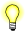This version of this document is no longer maintained. For the latest documentation, see http://www.qnx.com/developers/docs.

# log(), logf()

Compute the natural logarithm of a number

## Synopsis:

```#include <math.h>

double log( double x );

float logf( float x );```

## Arguments:

x
The number that you want to compute the natural log of.

## Library:

libm

Use the -l m option to qcc to link against this library.

## Description:

The log() and logf() functions compute the natural logarithm (base e) of x:

loge x

A domain error occurs if x is negative. A range error occurs if x is zero.

## Returns:

The natural logarithm of x.If an error occurs, these functions return 0, but this is also a valid mathematical result. If you want to check for errors, set errno to 0, call the function, and then check errno again. These functions don't change errno if no errors occurred.

## Examples:

```#include <stdio.h>
#include <stdlib.h>
#include <math.h>

int main( void )
{
printf( "%f\n", log(.5) );

return EXIT_SUCCESS;
}```

produces the output:

`-0.693147`

## Classification:

Safety:
Cancellation point No
Interrupt handler No
Signal handler No